## Matrix Multiplication

The productof two Matricesandis defined by(1)

where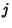is summed over for all possible values ofand. Therefore, in order for multiplication to be defined, the dimensions of the Matrices must satisfy(2)

wheredenotes a Matrix withrows andcolumns. Writing out the product explicitly,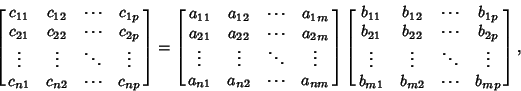(3)

whereMatrix multiplication is Associative, as can be seen by taking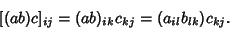(4)

Now, since,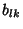, andare Scalars, use the Associativity of Scalar Multiplication to write(5)

Since this is true for alland, it must be true that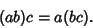(6)

That is, matrix multiplication is Associative. However, matrix multiplication is not, in general, Commutative (although it is Commutative ifandare Diagonal and of the same dimension).

The product of two Block Matrices is given by multiplying each block(7)